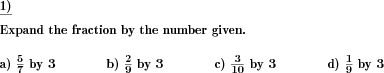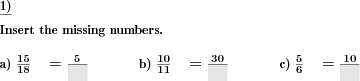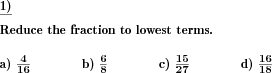Custom math worksheets at your fingertips# Details for problem "Convert fractions to like fractions"

Quickname: 6760

Elementary School, Primary School, Junior High School, Middle School, High School.

## Summary

Convert multiple fractions to like quantities

## Example## Description

Converting functions to like fractions is compulsory for learning further concepts of fractions. A set of fractions is presented. They are real and fully truncated fractions. All the fractions have different denominators. The task is to make the fractions all alike.

This sort of problem can be used as a small or whole group activity or independent/ homework practice additionally. The complexity level can be personalized to use these worksheets from grades 6 and 7. Exercising these tasks will offer an expansion of the following skills.

1. Revise the concept of LCM.
2. Recall identification of fractions as like or unlike fractions.
3. Learn to use LCM for converting fractions to like fractions.
4. Describe the process to convert fractions to like fractions.
5. Build strong concepts of like fractions to be used in the comparison, ordering, and other arithmetic concepts of fractions.

Customize these worksheets to achieve differentiated learning outcomes.

• The number of fractions is adjustable from 1 to 4.
• The number space from which both numerator and denominator are chosen is selectable. The main denominator of the fractions with the same name will also be in this number space.
• The number range starts from 20 to 100000.
• The number of problems is also adjustable from 1 to 10.

The answer key contains all sets of fractions converted to like terms.

Download free printable worksheets for this math problem here. The worksheet contains the problems only, the solution sheet includes the answers. Just click on the respective link.

•Worksheet 1Solution sheet with answers
•Worksheet 2Solution sheet with answers
•Worksheet 3Solution sheet with answers

If you can not see the solution sheets for download, they may be filtered out by an ad blocker that you may have installed. If this is the case, please allow ads for this page and reload the page. The solution sheets will then reappear.

• Do these sample worksheets do not really fit?
• Do you need more math worksheets, with a different level of difficulty?
• Would you like to combine different problems on a worksheet and adjust them to your needs?
• As a teacher, you can put together your own worksheets using the automatically generated math problems provided.
With a free initial credit, you can start creating your own math worksheets in a few minutes.

You can try it for free! Register here, to create custom worksheets now!

## Customization options for this problem

Parameter
Possible values
Number of problems
1, 2, 3, 4, 5, 6, 7, 8, 9, 10
Number range
20, 30, 40, 50, 100, 200, 500, 1000, 10000, 100000
Number of fractions
1, 2, 3, 4

## dw-Math worksheet templates that do contain this problem

Name
Title
Description
Reducing and expanding fractions
Expansion and reduction of fractions, and converting fractions to like quantities.

## Other types of problems that appear on worksheets with this problem:

Relevance
Name
Description
Quickname
Example
****
Expand fractions by given number
Fractions are to be expanded by a given number****
Missing elements in unlike fractions
In unlike fractions of the same value, numerators or denominators have to be filled in.****
Reduce fractions
A fraction has to be reduced to lowest terms.